## 1.

The Correct Answer is (E) — Recall that congruent means that two polygons are the same shape and size. Since ∆ABC and ∆ACD are congruent, you know that each angle from one triangle corresponds to an angle of the other triangle. If you are having a hard time visualizing the triangles matching up, mark up the given diagram or draw your own to show the triangles side by side: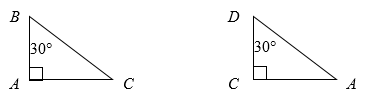Just by filling in the angles, you can see that the remaining angle in each triangle must be 60°. To find ∠BCD, simply sum ∠BCA and ∠ACD to get a total of 60° + 90° = 150°.

## 2.

The Correct Answer is (J) — The perimeter formula for a rectangle is 2l + 2w, because rectangles have two pairs of congruent sides and perimeter is simply their sum. Once you have the formula down, you can solve for the remaining side:

Now that you know the length of the longer and shorter side, finding the area is only a matter of multiplying the two together: wl = 250 meters × 350 meters = 87,500 square meters.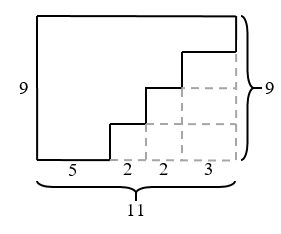Alternatively, you know that one of the longer sides is 350 meters, so the area can’t be larger than 350 × 350 = 122,500 square meters. The only answers that are less than this maximum value are (J) and (K). (K) is much too small to be a possible area, so you have found the correct answer, (J), with none of the algebra.

If you chose (K), you probably found the length of the shorter side and stopped there.

## 3.

The Correct Answer is (C) — All of the line segments intersect at right angles to each other, so the resulting perimeter of the shape is the same as a rectangle with the same dimensions: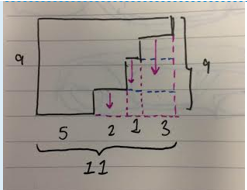Since one side has a length of 9 millimeters, you know that the opposite side also has a length of 9 millimeters. To find the length of the bottom side (which will be the same as the top) sum all the line segments parallel to it: 5 + 2 + 1 + 3 = 11 millimeters. Therefore, the perimeter of the entire figure is 2(9) + 2(11) = 40 millimeters.

## 4.

Since and are parallel lines intersected by two transversals, you know that the alternate interior angles are congruent. To find angle ∠DFG, look for its corresponding alternate interior angle, ∠ADF, given in the question as 50°.

Angles ∠BFE, ∠BFD, and ∠DFG all intersect at point F on a straight line, , so they are supplementary angles, meaning they add up to 180°. Inputting the expressions given for the two unknown angles, you can write out an equation solving for x:

## 5.

The Correct Answer is (B) — This question might seem tricky at first, because it is asking you to measure distances with units of time. However, in this case the only difference between time and any other variable is that you need to remember that one hour has 60 minutes. When translating the information given to you, think of the diameter of the circle as “2 hours long,” and solve for circumference by using the formula 2πr, or πd, where d is the diameter:

Circumference = πd = 3.14 × 2 = 6.28 hours

To convert the 0.28 hours into minutes, use a conversion factor: . This leaves you with a final answer of 6 hours and 17 minutes.

If you got (C), you probably forgot to convert the leftover 0.28 hours to minutes, and left it as is.

## 6.

The Correct Answer is (K) — It may be helpful for you to label congruent angles on the diagram so that checking the answer choices becomes quicker: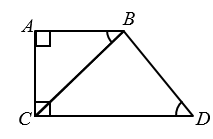You can eliminate (F), because ∠ABC and ∠BCD are alternate interior angles, formed by the transversal intersecting parallel lines and .

You can also eliminate (H), because ∠BDC and ∠BCD are opposite the two equal legs of an isosceles triangle so they are congruent as well.

If ∠BDC = ∠BCD, and ∠ABC = ∠BCD, then ∠BDC must also be equal to ∠ABC, eliminating answer choice (G).

Finally, you can eliminate (J), because ∠ACD and ∠CAB are both right angles formed by the line , which is perpendicular to the two parallel lines it crosses.

This leaves you with only one answer choice, (K). These two angle pairs are not congruent because, following from our above analysis, ∠ACB is 90° – ∠BDC. The two would only be equal if they were both 45°, and you cannot make that assumption.

## 7.

The Correct Answer is (C) — This question requires you to recall your knowledge of proportions and scale factors. You can see that the ratio of the sides of the poster, 12:18, is equal to the ratio of the sides of the original picture, 4:6, multiplied by a scale factor of 3. If the poster is 3 times the original picture, then the basketball net will be 3 × 3 = 9 inches tall. However, the question asks how tall it is in feet, so you need to use a conversion factor. Thankfully, this is given to you in the question: .

If you chose (E), you probably left the height of the basketball net in inches.

If you picked (D), you probably divided the original height by 3 instead of multiplying.

## 8.

The Correct Answer is (G) — If you have a hard time visualizing the overlap of line segments and , don’t be afraid to label the diagram given to you: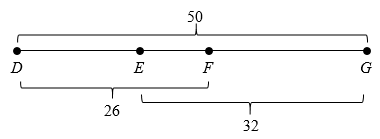Marking the diagram makes it clear that, if you subtract the length of from the sum of the two overlapping line segments, you get the overlap itself, : (32 + 26) – 50 = 58 – 50 = 8 units.

## 9.

The Correct Answer is (B) — The formula for the area of a square is simply its side length squared, so you can square-root the area to work backwards and find its side length: units. The square shares a side with an equilateral triangle, so you know that each side of the triangle is also 8 units long. The perimeter of a triangle is the sum of its sides, so 8 × 3 equal sides = 24 units.

If you got (E), you probably thought the side length of the triangle was 64 units and misread the question.

## 10.

The Correct Answer is (G) — Because you are dealing with a right triangle, you can use the Pythagorean Theorem to solve for the missing side lengths in terms of x, and thus find the value of x:

You may have noticed no ± sign in front of the square-root of 27. It is true that when you square-root both sides of an equation you generate two possible solutions, but you can ignore the negative one in this case because there is no way for a side to be negative inches long!

If you chose (K), you probably forgot to square-root the value for x2.

## 11.

The Correct Answer is (A) — For this question, you may find it helpful to first sketch out what her garden might look like: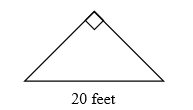The area of a triangle is given by the formula . To find the height of this triangle, draw a line perpendicular to the base from the opposite vertex. You know that this line splits the base in half because it is an isosceles triangle, so you are left with: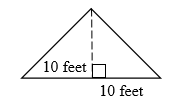If you cut an isosceles right triangle in two, you get two identical isosceles triangles, so you know that their two bases are each 10 feet. From the diagram, you can easily see that the height is 10 feet. The area is square feet.

## 12.

The Correct Answer is (K) — Similar triangles are triangles that are almost congruent, where one triangle is simply the other multiplied by a scale factor. Since the angles and ratios of the sides will remain the same between triangles, you can compare them side-by-side to find the scale factor: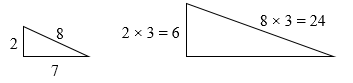You have found that the larger triangle is the smaller triangle scaled up by a factor of 3. If the scale factor was not obvious from the diagram, you can divide a side in the longer triangle by its corresponding side in the smaller triangle for the same result: . Therefore, the perimeter of the larger triangle will be 3 times the perimeter of the smaller triangle: 3 × (2 + 7 + 8) = 3 × 17 = 51.

If you chose (F), you found the perimeter of the smaller triangle.

## 13.

The Correct Answer is (B) — First, translate the relationships that the question describes to you in words to construct a system of equations relating the balloons’ volumes:

Subbing the value of VA from the second equation into the first, you can then replace the V with the formula for volume of a sphere:

Finally, plug in the value for the radius of B, and solve:

## 14.

The Correct Answer is (J) — If a man creates a right triangle with sides of 5 and 12 inches, you should instantly recognize that this is a 5:12:13 special triangle and that the remaining side—the diagonal—must have a value of 13 inches.

Failing to remember your special triangles, you could draw out the pizza and solve for the diagonal using the Pythagorean Theorem: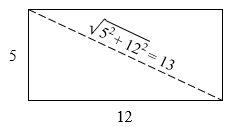## 15.

The Correct Answer is (C) — Recall that the number of lines of symmetry in a regular polygon is equal to the number of vertices the polygon has. Therefore, the number of lines of symmetry A has is 5, and the number B has is 3, so A has 2 more lines of symmetry than B. If you aren’t sure that the number of lines of symmetry = number of vertices, you can draw in the lines and count them manually to arrive at the same conclusion: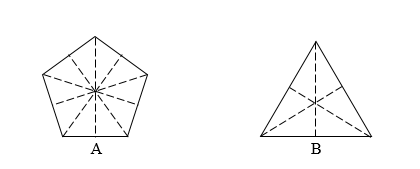If you got (A), you probably just found the number of lines of symmetry A has and stopped there.

## 16.

The Correct Answer is (F) — Since each of the squares has the same area of 361 m2, you know that they are all congruent. Remember that the area of a square is equal to its side length squared, so working backwards, each square has side lengths of meters. Points E, C, and G are in the middle of the first, second, and third square respectively, so it follows that they bisect the sides of these squares; each of their overlapping lengths are therefore 9.5 meters. Labelling this on the diagram, it becomes clear that each “corner” piece has the same length of one of the sides, 19 meters: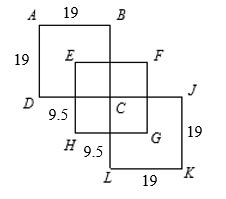To find the perimeter of this shape, sum the number of full side lengths and half-side lengths: (4 × 19) + (8 × 9.5) = 76 + 76 = 152 meters.

## 17.

The Correct Answer is (C) — Recall that the area of a parallelogram is equal to base × height. Reading these off of the diagram, you can plug them into this formula to get an area of 20 × 12 = 240 square units.

## 18.

The Correct Answer is (J) — This question requires you to use your knowledge of ratios and proportions. The ratio of angle a to 360° (the angle of a full circle) is equal to the ratio of the sector’s area to the area of the circle. Writing this out, you can solve for a without even needing to calculate the area of the circle:

## 19.

The Correct Answer is (D) — The question tells you that a hexagon is made up of 6 congruent equilateral triangles, so you know the area of the hexagon is 6 times the area of one of the triangles. Since the hexagon has edges that are 4 units long, you know that one of the triangles looks like this: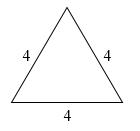The area of a triangle is , so to find the area, first you need the height. Here, the height is represented by the dotted line: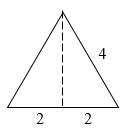Because you are dealing with an equilateral triangle, the height will bisect the base. Thus, you can solve for it using the Pythagorean Theorem:

Therefore, the area of one triangle is equal to units squared, and the area of the whole hexagon is units squared.

## 20.

The Correct Answer is (J) — To solve this question, you may find it helpful to sketch out the shelf with a box resting on it. There are three possible orientations for the boxes depending on how you lay it down on the shelf. The most space efficient orientation is more apparent once you draw these scenarios. With the shortest, 6”, side of the box lined up against the longest, 48”, side of the shelf, you can fit boxes on the shelf: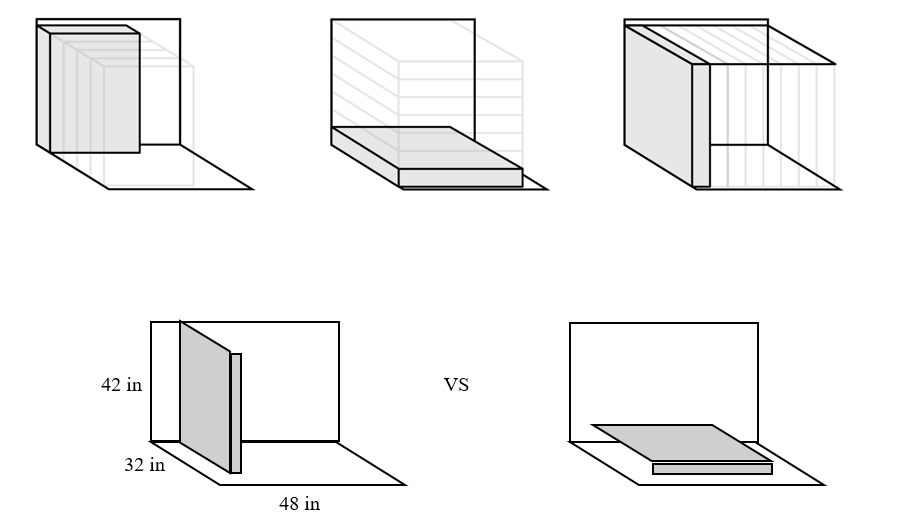If you chose (F), you aligned the 6” side of the box with the 32” side of the shelf, an orientation that can only fit boxes.

If you chose (H), you aligned the 6” side of the box with the 42” side of the shelf, a scenario that would fit only boxes.